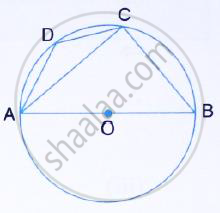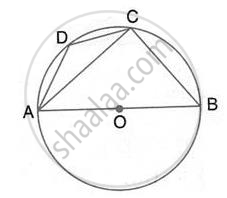Share

# Abcd is a Cyclic Quadrilateral in a Circle with Centre O. If ∠Adc = 130°; Find ∠ Bac. - Mathematics

Course

#### Question

ABCD is a cyclic quadrilateral in a circle with centre O. If ∠ADC = 130°; find ∠ BAC.#### SolutionHere ∠ACB = 90°
(Angle in a semicircle is right angle)
Also,  ∠ABC = 180° -∠ADC = 180° - 130° = 50°
(pair of opposite angles in a cy clic quadrilateral are supplementary)
By angle sum property of right triangle ACB,
∠BAC = 90° - ∠ABC = 90° - 50° = 40°

Is there an error in this question or solution?